# Distro screenshots: Math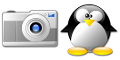Math: Solving linear equations with Mathomatic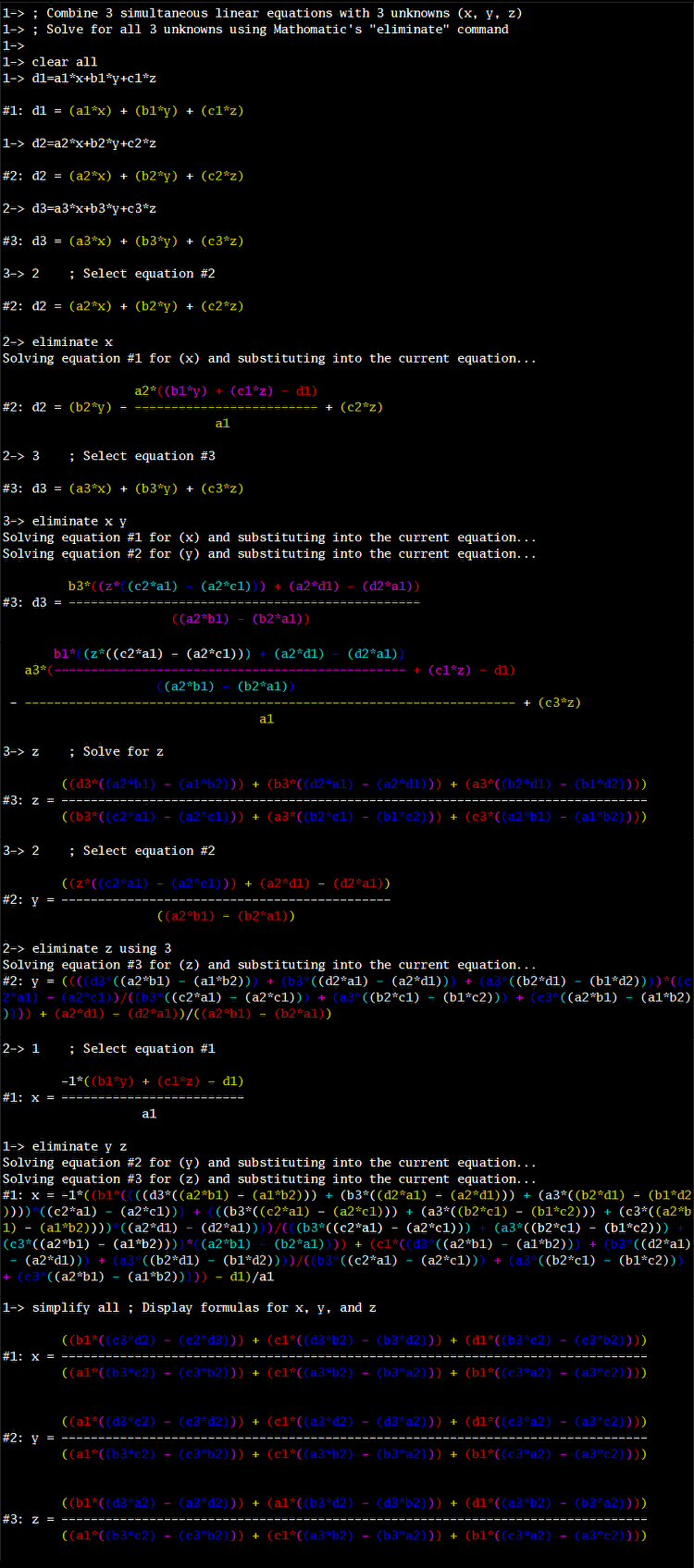QTStalker is a technical analysis tool. The program seems to be orphaned but it offers some useful features.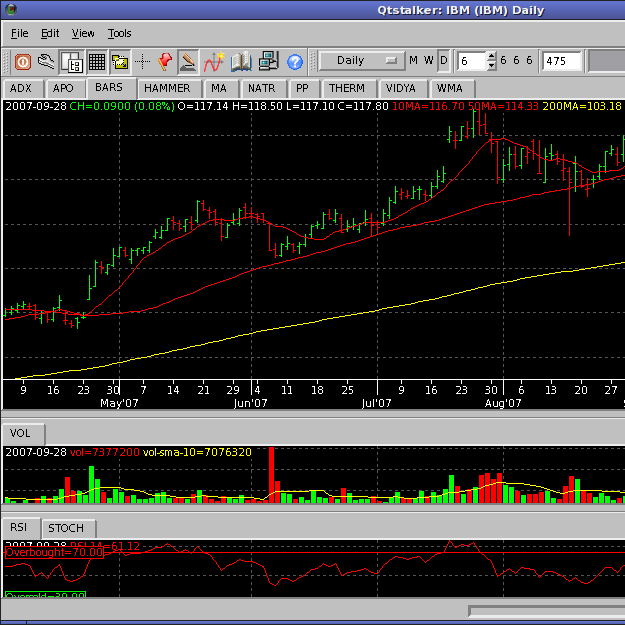Math: Octave

Octave is similar to Matlab®.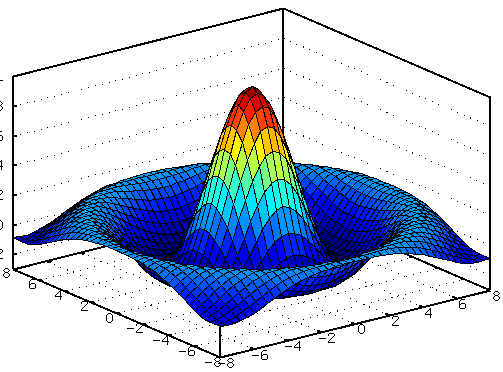Math: R

R is a statistics-oriented language.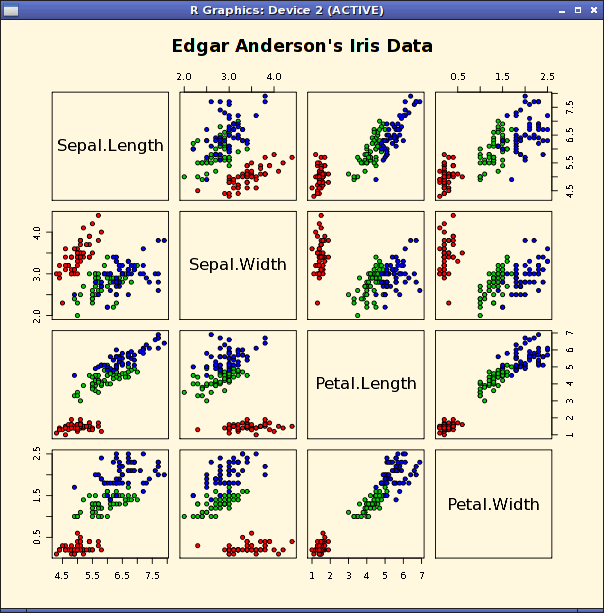Math: Designing a finite state machine with Qfsm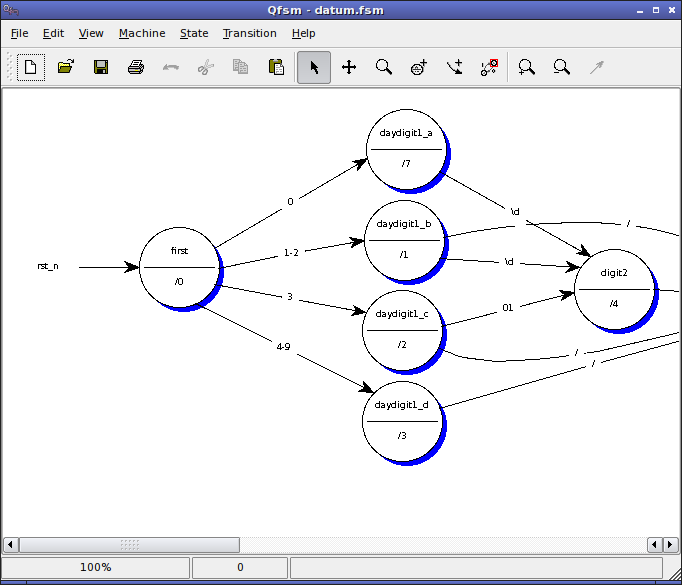Math: XaoS

XaoS is a fractal browser.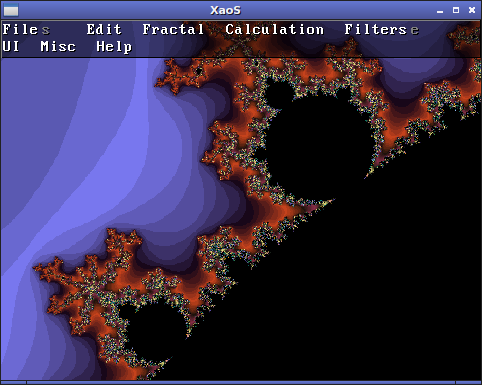Math, Education, Games: GNumch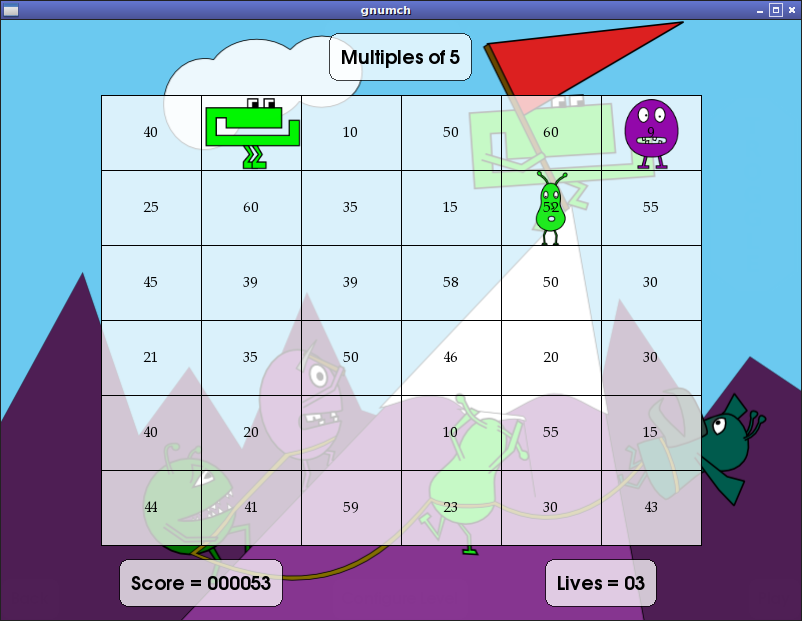Math: A few calculators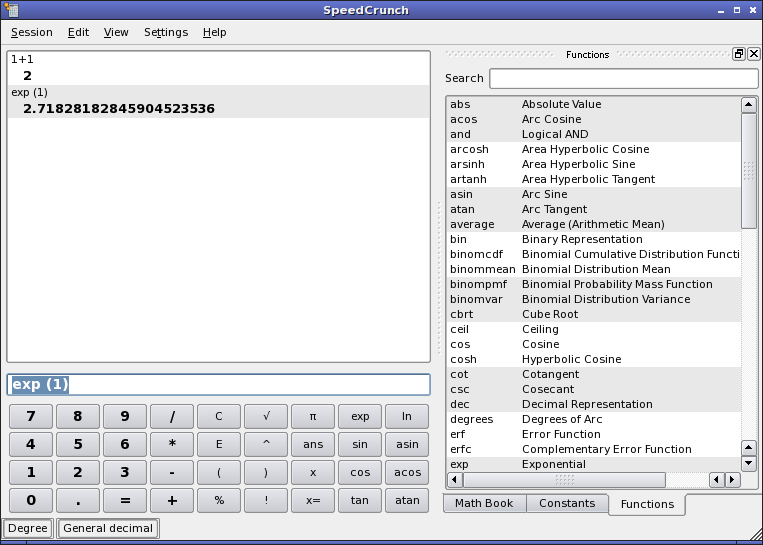SpeedCrunch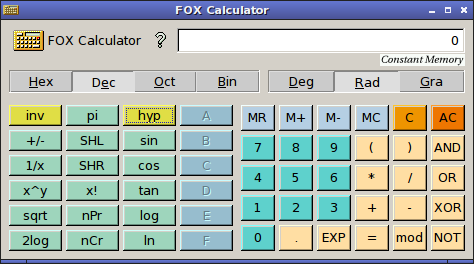FoxCalc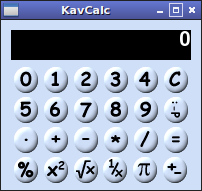KavCalc

Math: Every engineer needs a slide rule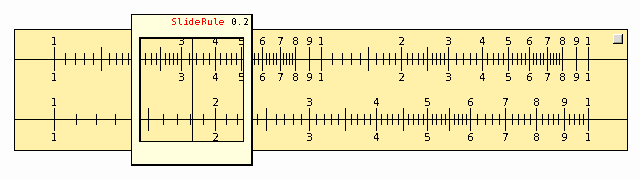Hosting provided by Zymic.Valid XHTML 1.0